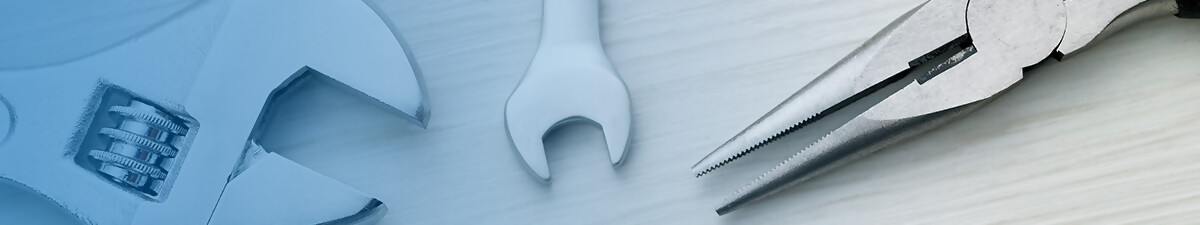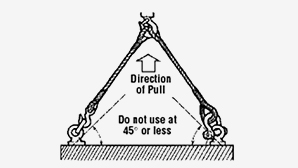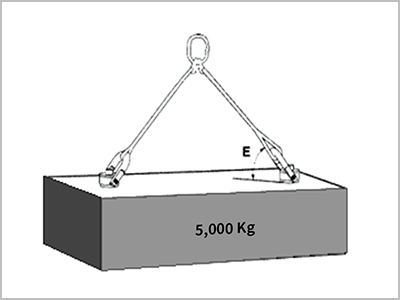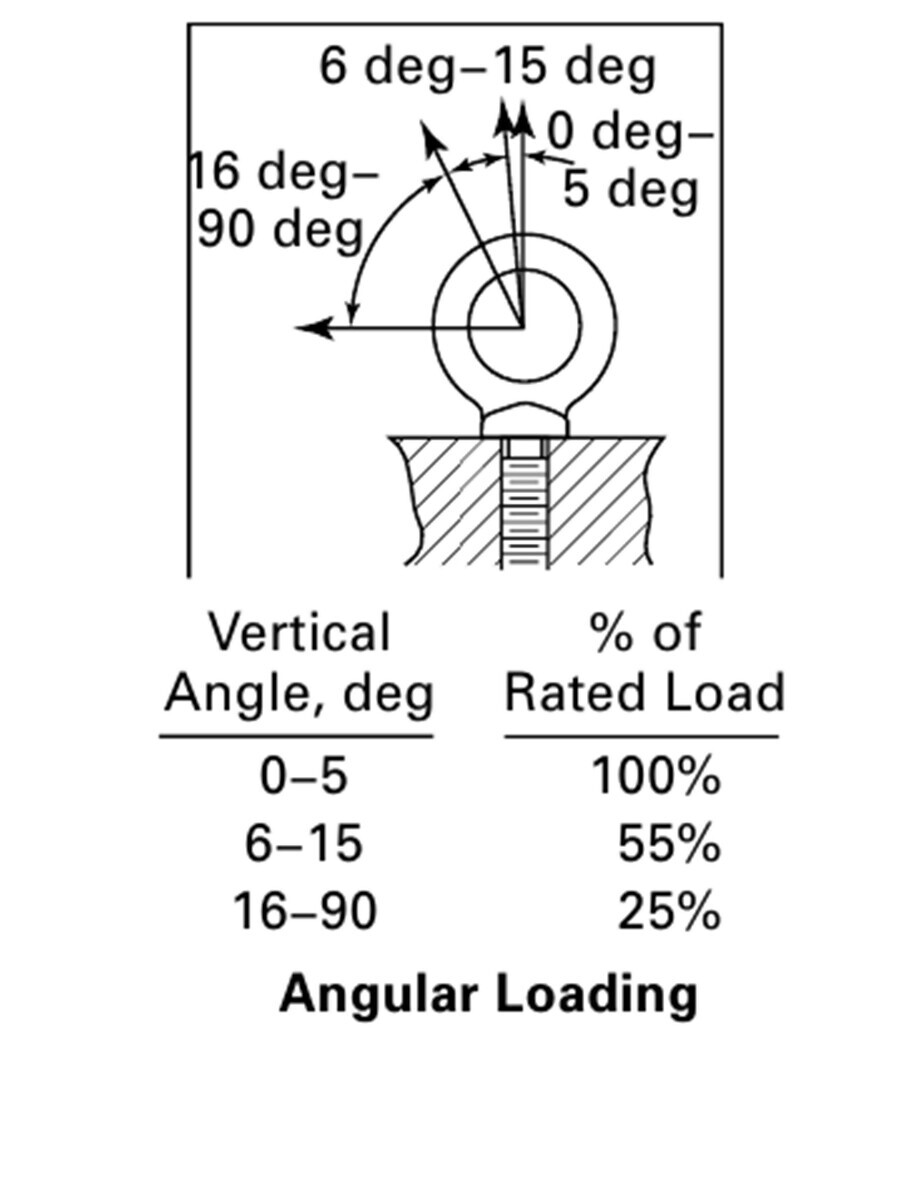#•Safety & Technical

> Safety and Technical > Formula & Precautions

### Formula & Precautions

Formula & Precautions

Hoist Ring  Formula & Precautions

Hoist Ring Formula

1. Hoist rings have a safety factor that is more than 5 times greater than that of Eye-bolts.Unless raised in perfectly vertical directions, eye-bolts’ (which are widely used in the field today) rated load ability tends to decline sharply.

For example, let’s assume that we are going to use two eye-bolts that each have a rated load ability of one (1) metric ton and each eye-bolt is lifted at an angle of 45 degrees in relation to the center lifting chained device (see the figure). Each eye-bolt will be able to withstand a load of only 250 kilograms (Kg). This means the load ability of eye-bolt decreases by 75% at an angle of 45 degrees.

However, when using a hoist ring and the inclination angle of 45 is applied, the tensile strength of the hoist ring, which is one (1) metric ton, is reduced to only about 30% of its original tensile strength. Meaning, the hoist ring will have a tensile strength of about 700 kilograms per ton at 45 degrees. This means that hoist ring has a tensile strength that is 2.8 times higher than eye-bolt at the same inclination angle of 45 degrees.

2. By using hoist rings, you only need smaller sized (diameter) screws that are needed to be machined on the object to be lifted. It can be reduced by 25% when eye-bolts are used.

As described above, it has been confirmed that the rated load ability of the hoist ring is, at the very least, 2 to 3 times stronger than eyebolts in regards to tilted angle lifting. Due to the sheer difference in lifting ability, the size of the screws and threaded taps on various objects will have major differences when compared.

The threaded tap on an object should be matched with the threaded shank of the hoist ring (male thread), which is machined into an object necessary for fastening objects to be lifted. For example, if the weight of the product to be lifted is about 5 tons (5,000 KG) and two eyebolts or two hoist rings are used, the calculation of how the size of the screw tab should change when trying to lift objects at 45 degrees is as follows.

The following is a formula to figure out size and number of hoist rings to lift load(s). The load on each hoist ring is not just the total weight divided by the number of hoist rings. The load can be greater at lower angles.W = 5,000 Kg

Formula: L 1 = W / N Sin E

L 1 = Load on each Hoist Ring
N = Number of Hoist Rings
E = Lifting angle

If  E = 45 º
L 1 = (5,000 / 2 x Sin 45 º)
= (5,000 / 2 x 0.70711)
= (5,000 / 1.414)
= 3,536.07 Kg

L 1 = 3,536.07 Kg

According to calculations, each installed hoist ring on the object has a load of 3,536 Kg. Therefore, when hoist rings are selected as lifting devices, the diameter of the screw of the hoist ring should be approximately M24 (screw diameter is 24 millimetres).

On the other hand, if you use eye-bolts at an angle of 45 degree lifting,L 2 = Load on each eye-bolt at 45 degree
= ( W x 0.25 )

L 2 = (5,000 Kg  x 0.25)
=  1,250 Kg

According to calculations, each installed eye-bolt on the object has a load of about 1,265 Kg(L 2) at the angle of 45 degree lifting. Therefore, when eye-bolts are selected as lifting devices, the diameter of the screw of the eye-bolt should be approximately M30 (screw diameter is 30 millimetres).

In other words, if you choose eye-bolts as lifting devices, it concludes that you need to machine screw tab on the object 25% larger than when you choose hoist rings as your lifting devices. This also means you need to use eye-bolts with much larger screw diameter unnecessarily.

Hoist Ring Safety precautions

· Never exceed Rated load. The safety factor is required in case of misuse such as overload or uneven loads.
· Never install hoist rings on uneven planes.